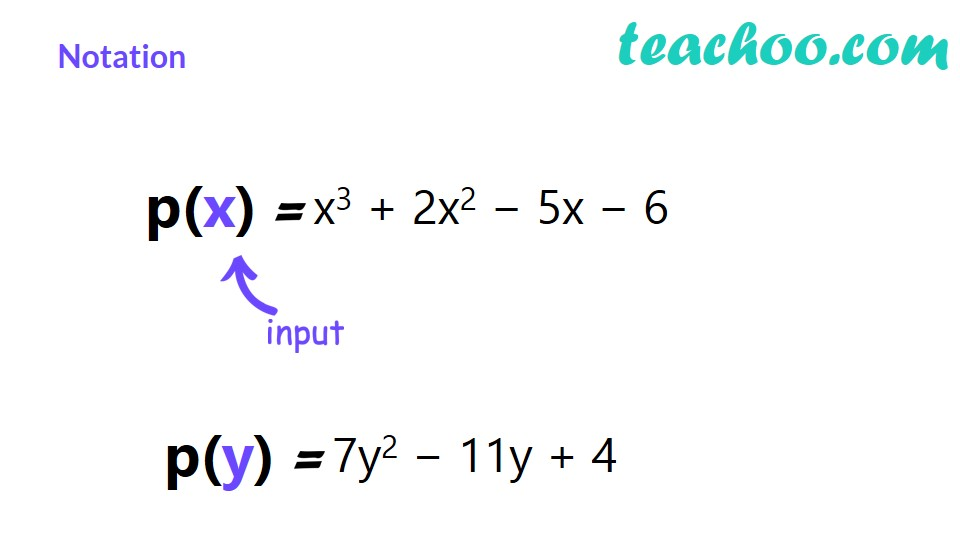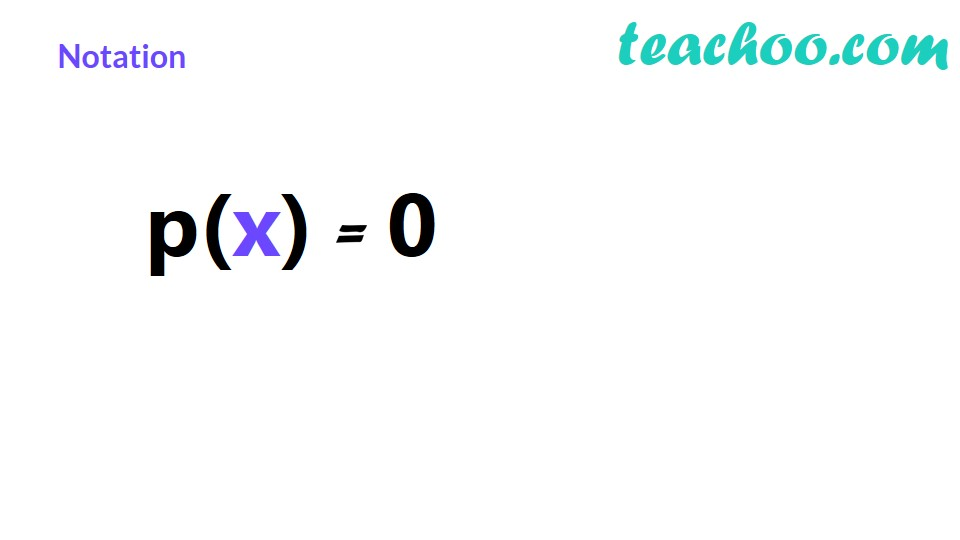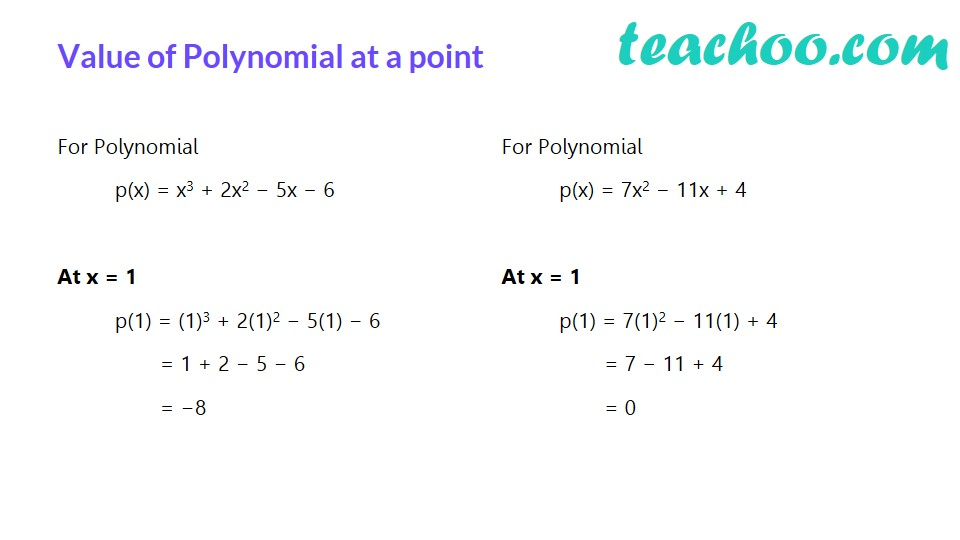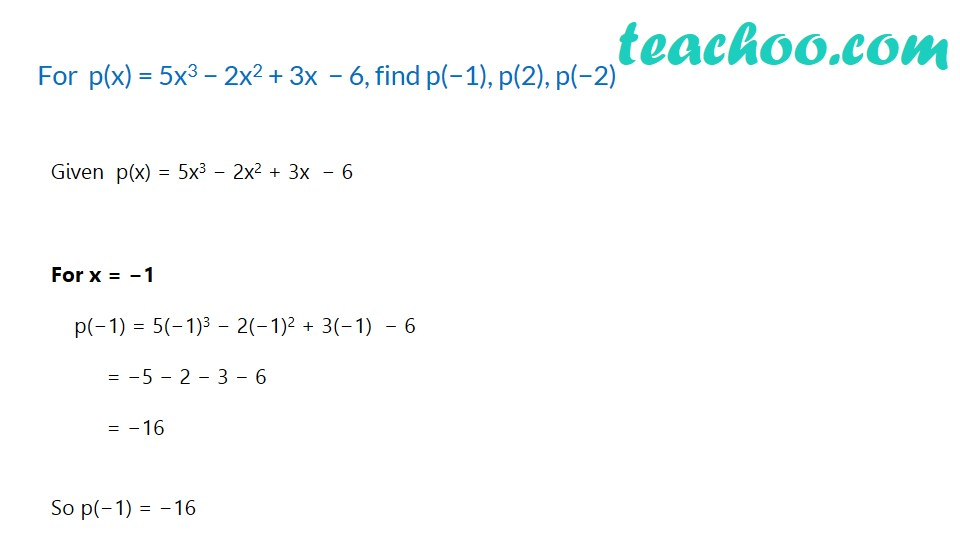1. Chapter 2 Class 9 Polynomials (Term 2)
2. Concept wise
3. Value of a polynomial at a given point

Transcript

Notationp(x) x3 + 2x2 − 5x − 6 p(y) 7y2 − 11y + 4 Notationp(x) Value at a pointValue of Polynomial at a pointFor Polynomial p(x) = x3 + 2x2 − 5x − 6 At x = 1 p(1) = (1)3 + 2(1)2 − 5(1) − 6 = 1 + 2 − 5 − 6 = −8 For Polynomial p(x) = 7x2 − 11x + 4 At x = 1 p(1) = 7(1)2 − 11(1) + 4 = 7 − 11 + 4 = 0 Let’s do an example…For p(x) = 5x3 − 2x2 + 3x − 6, find p(−1), p(2), p(−2)Given p(x) = 5x3 − 2x2 + 3x − 6 For x = −1 p(−1) = 5(−1)3 − 2(−1)2 + 3(−1) − 6 = −5 − 2 − 3 − 6 = −16 So p(−1) = −16

Value of a polynomial at a given point